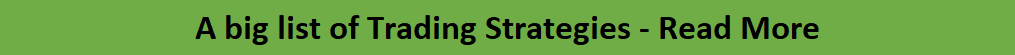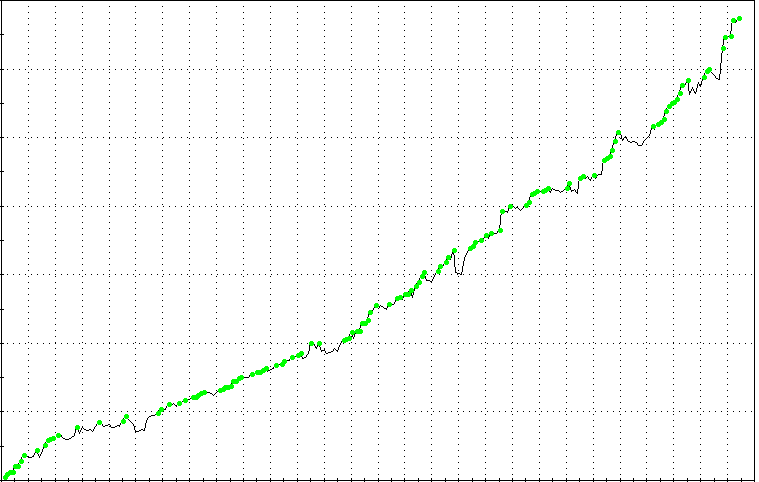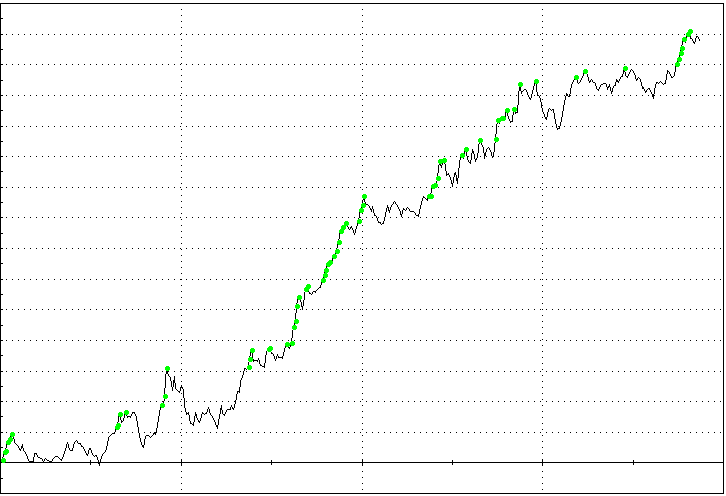Since 2013

• 100% Quantified, data-driven and Backtested
• We always show our results!
• Signals every day via our site or email
• Cancel at any time!

# What Is the Best Volatility Indicator?

Last Updated on 20 April, 2023 by Samuelsson

Market volatility is like a double-edged sword. While there is a need for enough volatility to be able to make some money from the market, high volatility increases the risk of being stopped out. So, what is the best indicator for effectively tracking volatility?

There is a variety of indicators traders and analysts use to track volatility, but some are more suited for certain markets than others. The best volatility indicator is the one that suits the market you are trading and you are comfortable with. To know that you need to backtest, backtest, and backtest.

## Trading Strategy Membership Monthly Edges

\$42 per strategy

In this post, we will discuss what volatility is, the different types, and the various indicators for measuring volatility. In the end, we will try to discuss what the best volatility indicator should be.

## What is volatility and which one is the best?

Volatility is a term in financial trading that expresses the variability of price movement. It is basically a way of measuring the rate of price movement. A market is said to be volatile if it exhibits rapid fluctuations in price, and non-volatile or stable if it has moderate price fluctuations.

The natural rhythm of price movement is to swing up and down whether it is trending (having an overall upward or downward direction) or not (moving sideways), but volatility considers the size and speed of those swings. When a market is volatile, it has huge and wild price swings, which makes it difficult to trade. You often see such after certain data releases or when there is news about important political or social events or some serious natural disasters.When the market is quiet (low volatility), the price tends to move more slowly, and the swings are milder, with smaller price bars. For intraday traders, in the forex market, you can see that during the Asian session, but in the stock market, you see it during lunchtime.

Different market situations require different approaches. Periods of high volatility are good for breakout strategies and scalping, while periods of low volatility may be suitable for a more relaxed trading approach. Knowing what phase the market is in will assist you in determining the best approach to use in the market, which is why you need to know the indicators you can use to track volatility. But first, let’s understand the different types of volatility data. To find out which one is the best volatility indicator you can scroll down a bit.

## Different types of volatility

You should know that there are different ways of looking at market volatility. When market analysts and traders talk about volatility, they may be saying slightly different things, depending on the angle they are looking at it from. But the different interpretations do not change the basic definition of volatility, which is the rate at which a market moves.

Having said that, these are the various types of volatility based on how people interpret it:

• Historical volatility: This is calculated from actual price changes.
• Future volatility: It expresses the unknown rate at which a market will move going forward.
• Forecast volatility: This is an estimate of future volatility.
• Implied volatility: This volatility is calculated from changes in the options market.

## The common volatility indicators

Some of the common volatility indicators you may see out there include:

### Average true range (ATR)

Created by J. Welles Wilder Jr., the average true range is a technical chart indicator that measures price volatility. Although it was originally developed for the commodity market, the indicator can be applied to any other financial market, including stocks, exchange-traded funds, forex, bonds, and futures.

The indicator makes use of actual price data that have been printed in the past for its calculation, so it measures the historical volatility of the market in question. It does this by decomposing the entire range of an asset price for a period. The indicator calculates what the author called “true range” and then creates a 14-day exponential moving average (EMA) of that true range. It is plotted in the indicator box.

#### Calculating the ATR

In calculating ATR in a security, the first step is to find a series of price range values for that security. As you know, the price range of an asset for a given trading period is simply the high minus the low. The true range is taken as the highest value generated by one of the following:

• the most recent period’s high less the current low
• the absolute value of the most recent period’s high less the previous close
• the absolute value of the most recent period’s low less the previous close

The average true range is then got as an n-period exponential moving average of the true range. Generally, a 14-day moving average is used.

#### Interpreting the ATR

The indicator does not indicate the price direction; it only measures volatility caused by gaps and limit up or down moves. The average true range (ATR) was initially developed for commodities, but it is being used for other assets, such as stocks and indices.

The interpretation is simple: a high ATR indicates large trading ranges and therefore increased volatility, while low readings from ATR are generally consistent with periods of quiet or uneventful trading. To put it simply, a stock experiencing a high level of volatility will have a high ATR value, and a low volatility stock will have a low ATR value. The ATR was created to allow traders to measure, more accurately, the daily volatility of an asset by using simple calculations.

### The Bollinger Bands

The Bollinger Bands is a technical indicator that consists of three lines plotted over the price chart. It was developed in 1980 by the famous technical trader, John Bollinger, to help traders spot when a security is probably overbought or oversold, but because it makes use of mean price and standard deviation, it is an effective tool for estimating the level of volatility in the security.

Bollinger bands consist of three lines:

• The central line is a 20-period moving average which actually measures the mean price
• The upper band is a line plotted 2 standard deviations above the moving average
• The lower band is a line plotted 2 standard deviations below the moving average

As you can see, the indicator shows both the mean price and the 2 standard deviations away from the mean. Since standard deviation is a measure of variability (which is what volatility represents), the bands move in a way that reflects changes in volatility. When volatility is increasing, the bands expand, reflecting the increase in variability of the price data, and when volatility is decreasing, the bands constrict to reflect the reduction in variability.

#### Calculating the Bollinger Bands

Here is how the Bollinger Bands are calculated:

• Compute the simple moving average of the security in question, using a 20-day SMA which averages out the closing prices for the most recent 20 days by adding the newest data and dropping the oldest data.
• Calculate the standard deviation of the security’s price as new 20-day averages emerge
• Multiply that standard deviation value by two
• Both add and subtract that value from each point along the SMA to produce the upper and lower bands

#### Interpreting the Bollinger Bands

The Bollinger Bands indicator makes use of historical price data, so it estimates historical volatility. However, the indicator has many applications in trading, and estimating volatility is just one of them. The upper and lower bands create boundaries within which the price is expected to swing. When the price swings outside those boundaries, it is overextended — overbought (when it extends above the upper band) or oversold (when it extends below the lower band). On the aspect of volatility, the standard deviation is a measure of volatility, so when the markets become more volatile the bands widen, and during less volatile periods, the bands get squeezed.

### CBOE Volatility Index (VIX)

While the ATR and the Bollinger Bands measure historical volatility because they make use of past price data, the VIX measures the implied volatility, which is the volatility as expected by the market participants at the current moment. The VIX was created by the Cboe Global Markets — previously known as the Chicago Board Options Exchange (CBOE) — to reflect the market’s expectation of volatility in the S&P 500 Index. It is plotted as a chart of its own.

Also known by other names like the “Fear Gauge” or “Fear Index”, the VIX is used by investors, research analysts, and portfolio managers to estimate the level of risk and fear among participants in the U.S. stock market. The values of the VIX tells them how the big players are positioning against risk, which can give a clue about what is expected to happen in the equity market. So, it is simply a measure of market risk, fear, and stress.

#### Calculating the VIX

The VIX index is derived from the price inputs of the S&P 500 index options quoted on the Chicago Board Options Exchange. The values of the index are calculated using the Cboe-traded standard SPX options, which are to expire on the third Friday of each month, and the weekly SPX options that expire on all other Fridays.

The formula for VIX is mathematically complex, but this is how it works:

• It measures the expected volatility of the S&P 500 index by aggregating the weighted prices of several SPX puts and calls over a wide range of strike prices.
• Only qualifying options must have valid non-zero bid and ask prices that represent the market perception of which options’ strike prices will be hit by the underlying stocks during the remaining time to expiry.

#### Interpreting the VIX

Calculated from the prices of the S&P 500 index options, the VIX tries to estimate investors’ sentiments in the market by considering their risk management activities. Institutional investors go to the options market to hedge their exposure in stocks. When they become very active in the options market, the VIX increases, which shows that they are expecting an adverse reaction in the stock market.

## Other volatility indicators

There are other less-common volatility indicators, and they include:

• Chaikin Volatility
• Volatility Bands
• Volatility Ratio (Schwager)

### Chaikin Volatility

Not to be confused with Chaikin Oscillator which is the more popular indicator, this indicator calculates volatility as the ratio of change of the average High-Low difference to the average High-Low difference some n-period ago.

However, the problem with this method of measuring volatility is that in situations when there is a prolonged period of high volatility or low volatility, the Chaikin Volatility Oscillator would show near-zero value. Nevertheless, the indicator can be used for comparative volatility analysis. It is plotted in the indicator box.

### Volatility Bands

This is not a particular indicator. Many indicators are called Volatility Bands because they all use a similar principle to the Bollinger Bands. But instead of using a multiple of the standard deviation to create the bands, these indicators make use of other parameters of rate variability to create the bands.

They are available for some trading platforms as custom indicators. An example is Sylvain Vervoort’s Volatility Bands, which plots on the price chart as an overlay.### Volatility Ratio (Schwager)

This volatility indicator is calculated using the true range values of different periods. It is the ratio of the current true range to the exponential moving average of the true range.

In essence, it shows how the current true range compares to the true range of in the previous candles. It is a custom indicator that is plotted in the indicator box on the chart platforms.

## What is the best volatility indicator?

So, which volatility indicator is the best? Well, it depends on the market you are dealing with. If you are trading stocks or an ETF that tracks the S&P 500 Index, the CBOE VIX is your best bet. But if you are trading the currency market or commodity market, you may be better off with the ATR or Bollinger Bands. Those two indicators can be used across different markets but remember that they only tell you about the volatility in the past.

Apart from the original VIX, which is based on the S&P 500 options, there are now corresponding volatility indexes for different assets. In the currency market, for example, there are special versions of VIX based on currency options — JYVIX (Japanese yen), BPVIX (Great Britain pound), and EUVIX (euro). These are more advanced ways of measuring forex volatility, but they are not as accessible to a common trader as a simple technical indicator.

## \$42 Per Strategy